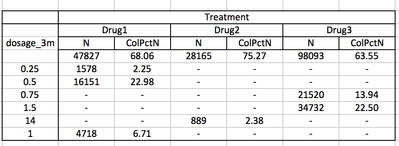## Proc tabulate calculate percentages by subgroups

I have the following table:Suppose to look at Drug3 dosage 0.75 and 1.5. Is there a way with proc tabulate or another proc to compute the percentage on 21520 + 34732? In other words, the percentage for 0.75 should be 21520/(21520 + 34732)=38% and for 1.5 should be 34732/(21520 + 34732)=61%

2 REPLIES 2Ksharp
Super User

## Re: Proc tabulate calculate percentages by subgroups

I think PROC SQL can do that.
Can you post your data and the output you want?

## Re: Proc tabulate calculate percentages by subgroups

If you do not want the missing values for your dosage_3m variable included in the denominators for Pctn calculations then you need to exclude them, such as not include MISSING on the class statement for that variable.

If you want to include the missing then you will have to modify the data and pick another approach to get something like this in proc tabulate. One approach would be to add another variable has a value of 1 only when Dosage_3m is not missing and then use that variable as a Var variable and ColPctSum with that variable instead of ColPctN of the Class variable.

From SAS Users blog